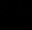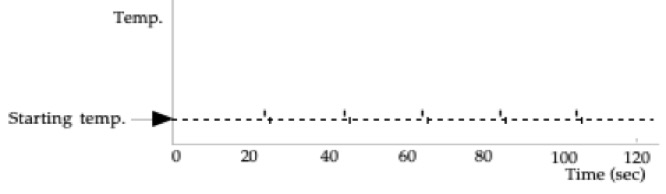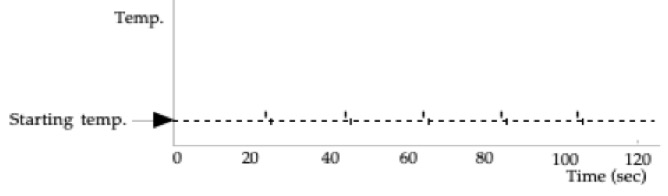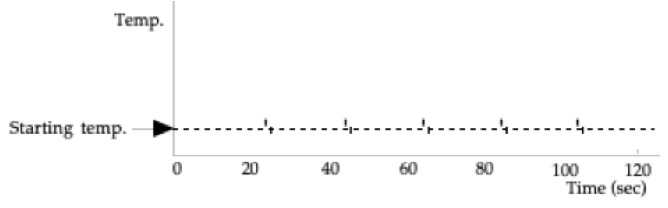Interactive Lecture Demonstrations

# Directions:  Click here to download the Prediction sheet on which you will record your predictions. Write your name at the top to record your participation in these demonstrations.  For each demonstration, write your predictions on this sheet before making any observations. You may be asked to send this sheet to your instructor.

 Demonstration 1:  100 grams of water is initially at room temperature in a perfectly insulated cup (no heat can leak in or out).  Heat energy is transferred to the water at a steady rate for 80 seconds, and then no more heat is transferred.  Sketch to the right your prediction for the graph of the temperature of the water as a function of time. Only after you have made your predictions, click here to download and view a video of the experiment. (Note that eachon the graph represents an equal quantity of heat transferred by a heating coil immersed in the water.) Then, click here to view the starting and final temperatures. Compare the resulting graph to your prediction and explain its shape. How is the change in temperature related to the quantity of heat transferred to the water?Record Temperatures Below: Starting Temperature: _______ Ending Temperature:  _______ Change in Temperature: _______ Calculation 1:  Using the actual starting and ending temperatures of the water, determine the amount of heat energy that was transferred to the water in this process.   The specific heat of water is 4186 J/kg Cº.                Heat Energy Transferred = Q1 = Only after you have made your calculation, click here to compare it. Demonstration 2:  50 grams of aluminum is added to 100 grams of water.  The aluminum and the water are both initially at room temperature in a perfectly insulated cup.  Heat energy is transferred to the system at the same steady rate as in the previous demonstration for 80 seconds, and then no more heat energy is transferred.  (Note that the specific heat of water is 4186 J/kg Cº and the specific heat of aluminum is 900 J/kg Cº.) Do you think that the change in temperature of the water plus aluminum will be greater than, less than or equal to the change in temperature in the previous demonstration? Only after you have made your predictions, click here to download and view a video of the experiment. Then, click here to view the starting and final temperatures. Compare the results to your predictions and explain any differences. Why is the temperature change smaller than in Demonstration 1?Record Temperatures Below: Starting Temperature: ______ Ending Temperature:  ______ Change in Temperature: ______ Calculation 2:  Using the actual starting and ending temperatures of the system, determine the amount of heat that was transferred to the water and aluminum in this process.   The specific heat of water is 4186 J/kg Cº and the specific heat of aluminum is 900 J/kg Cº. Heat Transferred = Q2 = Only after you have made your calculation, click here to compare it. Demonstration 3:  Now 50 grams of aluminum are used to replace 50 grams of water, so that we start with 50 grams of water and 50 grams of aluminum, both initially at room temperature in a perfectly insulated cup.  Heat energy is transferred to the water and aluminum at the same steady rate as in the previous demonstrations for 80 seconds, and then no more heat energy is transferred.  (Again, the specific heat of water is 4186 J/kg Cº and the specific heat of aluminum is 900 J/kg Cº.) Do you think that the change in temperature of the water plus aluminum will be greater than, less than or equal to the change in temperature in Demonstration 2 (where there was 100 grams water with 50 grams aluminum)? Do you think that the change in temperature of the water plus aluminum will be greater than, less than or equal to the change in temperature in Demonstration 1 (where there was only 100 grams of water)? Only after you have made your predictions, click here to download and view a video of the experiment. Then, click here to view the starting and final temperatures. Compare the results to your predictions and explain any differences. Why is the temperature change larger than in Demonstration 1? Demonstration 2?Record Temperatures Below: Starting Temperature: ______ Ending Temperature:  ______ Change in Temperature: ______ Calculation 3:  Now let’s assume that the same amount of heat energy will be transferred to this system as was transferred in the previous two demonstrations.  Since you probably found that these amounts were slightly different, first take the average of the two amounts of heat: Heat Transferred = Q3 = 1/2 (Q1 + Q2) Since this heat will be transferred to the water and the aluminum, we can write down an equation that will allow us to predict the theoretical change in temperature of our system.  The amount of heat energy gained by each part of the system will be of the form mc /\ T.  In the space below, write down an equation which sets Q3 equal to the heat energy gained by the water and aluminum, and then solve it for   /\ T:                            Q3 =               /\T =   How does the theoretical numerical answer you just calculated compare with the actual change in temperature of the system?  Can you think of a possible explanation for any discrepancy? Only after you have made your calculation, click here to compare it.